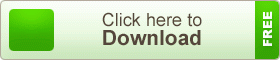# SSC Higher Mathematics Note 10th Chapter Binomial Expansion

SSC Higher Mathematics Note 10th Chapter Binomial Expansion. In our previous classes addition, subtraction, multiplication, division, square and cube related algebraic expression (Single term, binomial and polynomial) have been discussed. If the power of binomial or polynomial expression is more than three, in that case determining the value of such expression is laborious and time-consuming. If the power is more than three in which method the work is completed that will be presented in this chapter. Generally, the formula will be demonstrated for the power of n.

## SSC Higher Mathematics Note 10th Chapter Binomial ExpansionBy which the value of binomial expression of a non-negative integer of power will be possible to determine. But at this stage, the value of n will not exceed a definite limit n ≤ 8. A triangle will be introduce named as Pascal’s triangle so that students can easily understand and use the subject matter. The power of binomial expression may be a positive or negative integer or fraction. But our present discussion will be limited in an only positive integer of power. In the next classes, a detailed discussion will be included.

teachingbd24.com is such a website where you will get all kinds of necessary information regarding educational notes, suggestions and question patterns of schools, colleges, and madrasas. Particularly, you will get here special notes of physics that will be immensely useful to both students and teachers. The builder of the website is Mr. Md. Shah Jamal who has been serving for 33 years as an Assistant Professor of Physics at BAF Shaheen College Dhaka. He expects that this website will meet up all the needs of Bengali version learners /students. He has requested concerned students and teachers to spread this website home and abroad.

SSC Higher Mathematics Note